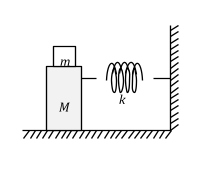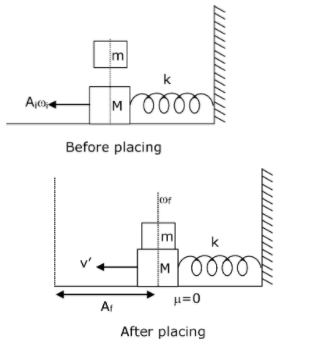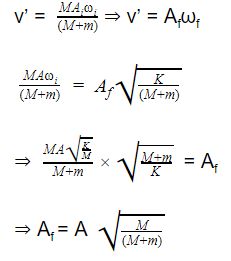# In the given figure, a mass M is attached to a horizontal spring which is fixed on one side to a rigid support. The spring constant of the spring is k. The mass oscillates on a frictionless surface with time period T and amplitude A. When the mass is in equilibrium position, as shown in the figure, another mass m is gently fixed upon it. The new amplitude of oscillation will be:a. $$A\sqrt{\frac{M}{M+m}}$$

b. $$A\sqrt{\frac{M}{M-m}}$$

c. $$A\sqrt{\frac{M-m}{M}}$$

d. $$A\sqrt{\frac{M+m}{M}}$$We know that ωf = $$\sqrt{\frac{k}{M-m}}$$ and ωi = $$\sqrt{\frac{k}{M}}$$ Ai = A

Also, momentum is conserved just before and just after the block of mass (m) is placed because there is no impulsive force. So

MAiωi = (M + m) v’(0)(0)